# The Pioneer Anomaly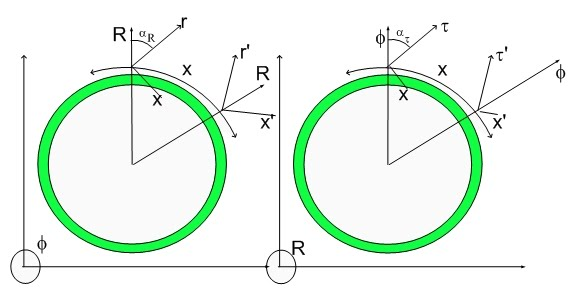The Pioneer Anomaly

People have been mesmerized by some extremely strange results... wait a second, I take it back...  Just a few nerds from NASA are really concerned about this.  These days, we are scared by the End of the World in the hands of CERN and the Large Hadron Collider...:)

This minor scientific puzzle refers to an unaccounted deceleration  observed on the trajectory of the Pioneer spacecraft on their way outwards our solar system.

There is a very small, very, really very small deceleration (8.5e-10 m/s^2), unaccounted after considering its distance from the Sun, interaction with planets, solar wind etc. The deceleration is very well defined and steady and can be seen in
the Pioneer Anomaly paper.

In the prior blog, after presenting the Hypergeometrical Law of Gravitation ...which corrects Newton and Einstein's works ... I suggested that this new equation would explain the Pioneer Anomaly...:)

Silly me...:)

Of course, I was wrong - this is a simpler problem.

The solution can be derived directly from the topology. It is our current understanding that only matter curves spacetime, this means that locally the curvature is quite well defined by the inexistence of localized matter between here and where the Pioneer spacecrafts are, thus rendering spacetime curvature equal to zero..:) that is, we believe that Pioneer spacecrafts exist in a locally flat space to a high approximation -of course, since this is an unaccounted decelerations, this statement cannot be taken absolutely.... that is the space curvature due to surrounding masses cannot explain.

Of course, if you consider our topology, it is clear that there is a curvature and that is equal to 1/R0. This is because in my theory, there is a fourth non-compact spatial dimension and our 3D Universe is curved in a lightspeed shockwave 3D Universe.

Below one can see two green circles representing two epochs (cross-sections on the 4D spatial manifold). The largest circle corresponds to our current time and B is our home on Earth. Light and matter travel at the speed of light within the expanding hypersphere. This means that light always travels along a diagonal path, oriented at 45 degrees off the perpendicular to the hypersphere. I am representing plane wavefronts reaching point B. It should be clear that different observed wavelengths will result from the observation of light from different epochs. This clearly illustrates the observed red shifting from peering into the sky and looking as far as you can. Hubble discovered this effect and proposed his famous Hubble Law. In the past, I corrected that law by explaining that it is a law applied to average velocities from the different epochs or regions of space.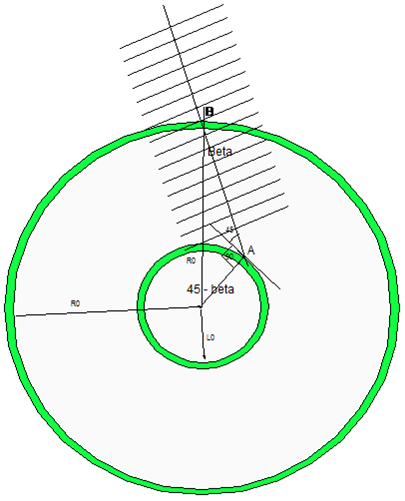Figure 1. Looking deep into the past.

Similarly, the wavelength of light depends upon what is the angle beta of observation. This is the physical reason for the red shifting.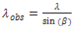This picture is quite telling. It shows the L0 radius of curvature of the time when the first stars lightened. At that time and at that radius of curvature, There was light…:)
It is important to notice that light always travels at 45 degrees with the Fabric of Space – 45 degree with the tangential line at point A. This is because light is modeled as a origin modulated dilaton field traveling together with the expanding hypersphere.

At the time of the Big Bang, Beta is zero and the distance AB is Ro or the radius of the Universe.

Now lest consider the near field problem, that is, light propagation among things close, in our neighborhood… solar system, galaxy… Under those conditions, beta is equal to 45 degrees and point A is almost right radially aligned with B. The two hyperspheres look like two hyperplanes and can be represented by the figure below: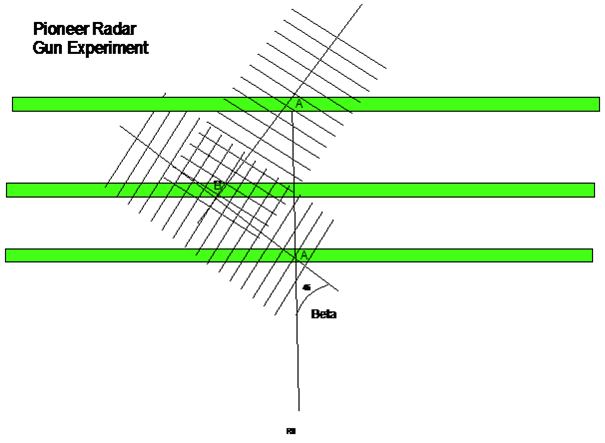Figure 2. Pioneer Radar Gun Experiment. Looking into a few minutes in the past.

I hope nobody would be silly enough to consider that I wrote radar gun experiment on a non tongue-in-cheek manner….:)

We can rewrite Hubble Law as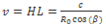L       (sorry the formula was missing L)

This also means that Hubble constant should depend upon how one calibrates it. Calibration is done using parallax or stellar candles for longer distances and the red shifts. These two calibrations are inconsistent since they look at different angles. One should use the near field calibration and then extrapolate it using the hypergeometrical argument.

Under near field conditions - relatively short distances with respect to the radius of the Universe -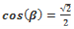.

Let's analyze how one measured the Pioneer deceleration.

Figure 2 shows what is happening according to the Hypergeometrical Universe model. Pioneer flies away in our 3D Universe represented by the Green Circles which is expanding at the speed of light radially.

Our measurements are modeled as if taking place in a flat 3D Universe. The curvature (1/R0) is not taken into consideration and that is the cause for the deceleration. As the craft travels at constant speed along the green curve, it travels a longer distance than the one traveled by light (shown as two straight lines at 45 degrees on Figure 2). This means that the light or radio measurement underestimates the actual distance and that underestimation increases as time goes by due to the curvature of 3D hypersphere. The difference in velocity is due to the centripetal acceleration and it is equal to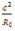. This acceleration (deceleration) has to b scaled by the underestimation factor of.

Thus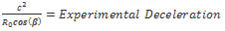.

The observed deceleration is 8.5e-10 m/s2, the speed of light = 299792458 m / s. This yields a value for R0= 15.82 Billion Light Years.

The actual age of the Universe is 15.82 Billion Years. This is the best measurement possible for the lifetime of the Universe. It doesn't depend upon the precise view of the furthest star.

As you can see, a very small and almost irrelevant piece of knowledge contained a key to a precise measurement of the lifetime of the Universe - something one could never hope to see with our very own eyes or instruments...

Cheers,

MP

PS- As expected, all pieces of the puzzle fit together and support the proposed topology and thus the Grand Unification approach. The moving shockwave creates an illusion of faster than the speed of light motion due to the light traveling within a moving framework (the hyperspherical lightspeed expanding 3D shockwave Universe). The wavefront would seem to be traveling at SQRT(2)*c from the absolute referential where we look at the Universe.

ONLY SPATIAL CURVATURE WOULD PROVIDE SUCH EFFECT CAN EXPLAIN THE EERY SUPER STEADY PIONEER DECELERATION..>:)

My gravitational law also has a negative component which depends upon v/c times the current gravitational acceleration. The spacecraft is likely to be traveling around 10-20 km/sec (Earth scape speed) which is around 10^8 the speed of light.  If the gravitational acceleration where the spacecraft where larger than 0.1 m/s^2 one would have to take that in consideration. Pioneer perceived deceleration is around 8.5 10^-10 m/s^2.  That is certainly not the case.
Current rating: 1

### New Comment

required

required (not published)

optional

required